# Spectral Factorization by the Wilson algorithm

John Burg (as interpreted by JFC)

jon@sep.stanford.edu

Newton's iteration for square roots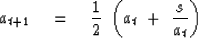(1)
converges quadratically starting from any real initial guess a0 except zero. When a0 is negative, Newton's iteration converges to the negative square root.

Quadratic convergence means that the square of the error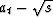at one iteration is proportional to the error at the next iteration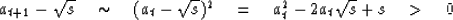(2)
so, for example if the error is one significant digit at one iteration, at the next iteration it is two digits, then four, etc. We cannot use equation (2) in place of the Newton iteration itself, because it uses the answer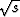to get the answer at+1, and also we need the factor of proportionality. Notice, however, if we take the factor to be 1/(2at), thencancels and equation (2) becomes itself the Newton iteration (1).

Another interesting feature of the Newton iteration is that all iterations (except possibly the initial guess) are above the ultimate square root. This is obvious from equation (2).

We can insert spectral functions in the Newton square-root iteration, for exampleand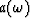.Where the first guess a0 happens to match,it will matchat all iterations. Something inspires Wilson to write the Newton iteration as(3)
and then express the spectrum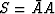as a Z-transform.(4)

Now we are ready for the algorithm: Compute the right side of (4) by polynomial division forwards and backwards and then add 1. Then abandon negative lags and take half of the zero lag. Now you have At+1(Z) / At(Z). Multiply out (convolve) the denominator At(Z), and you have the desired result At+1(Z). Iterate as long as you wish.

We show that At+1(Z) is minimum phase: Both sides of (4) are positive, as noted earlier. Both terms on the right are positive. Since the Newton iteration always overestimates, the 1 dominates the rightmost term. After masking off the negative powers of Z (and half the zero power), the right side of (4) adds two wavelets. The 1/2 is wholly real, and hence its real part always dominates the real part of the rightmost term. Thus (after masking negative powers) the wavelet on the right side of (4) has a positive real part, so the phase cannot loop about the origin. This wavelet multiplies At(Z) to give the final wavelet At+1(Z) and the product of two minimum-phase wavelets is minimum phase.

Claerbout is interested in factoring the Laplacian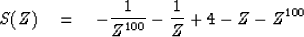(5)
It seems less confusing than the Kolmogoroff method, and so easier to teach. Furthermore, it might be better because we can think of upper and lower triangular matrices, instead of recursive filters. The advantage of the triangular matrices is that they embody time-variable filters.

Burg is interested in extending the Wilson algorithm to cross spectra.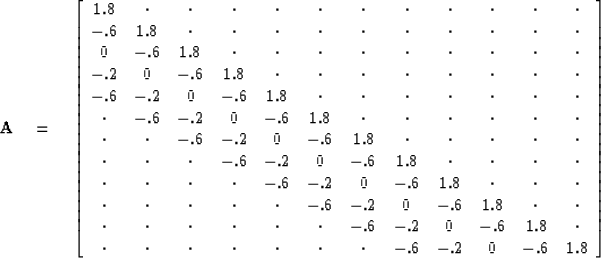(6)